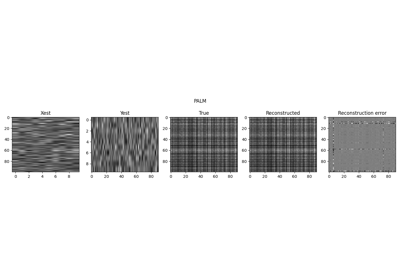# pyproximal.optimization.palm.PALM#

pyproximal.optimization.palm.PALM(H, proxf, proxg, x0, y0, gammaf=1.0, gammag=1.0, niter=10, callback=None, show=False)[source]#

Proximal Alternating Linearized Minimization

Solves the following minimization problem using the Proximal Alternating Linearized Minimization (PALM) algorithm:

$\mathbf{x}\mathbf{,y} = \argmin_{\mathbf{x}, \mathbf{y}} f(\mathbf{x}) + g(\mathbf{y}) + H(\mathbf{x}, \mathbf{y})$

where $$f(\mathbf{x})$$ and $$g(\mathbf{y})$$ are any pair of convex functions that have known proximal operators, and $$H(\mathbf{x}, \mathbf{y})$$ is a smooth function.

Parameters
Hpyproximal.utils.bilinear.Bilinear

Bilinear function

proxfpyproximal.ProxOperator

Proximal operator of f function

proxgpyproximal.ProxOperator

Proximal operator of g function

x0numpy.ndarray

Initial x vector

y0numpy.ndarray

Initial y vector

gammaffloat, optional

Positive scalar weight for f function update

gammagfloat, optional

Positive scalar weight for g function update

niterint, optional

Number of iterations of iterative scheme

callbackcallable, optional

Function with signature (callback(x)) to call after each iteration where x and y are the current model vectors

showbool, optional

Display iterations log

Returns
xnumpy.ndarray

Inverted x vector

ynumpy.ndarray

Inverted y vector

Notes

PALM  can be expressed by the following recursion:

$\begin{split}\mathbf{x}^{k+1} = \prox_{c_k f}(\mathbf{x}^{k} - \frac{1}{c_k}\nabla_x H(\mathbf{x}^{k}, \mathbf{y}^{k}))\\ \mathbf{y}^{k+1} = \prox_{d_k g}(\mathbf{y}^{k} - \frac{1}{d_k}\nabla_y H(\mathbf{x}^{k+1}, \mathbf{y}^{k}))\\\end{split}$

Here $$c_k=\gamma_f L_x$$ and $$d_k=\gamma_g L_y$$, where $$L_x$$ and $$L_y$$ are the Lipschitz constant of $$\nabla_x H$$ and $$\nabla_y H$$, respectively.

1

Bolte, J., Sabach, S., and Teboulle, M. “Proximal alternating linearized minimization for nonconvex and nonsmooth problems”, Mathematical Programming, vol. 146, pp. 459–494. 2014.

## Examples using pyproximal.optimization.palm.PALM#Low-Rank completion via Matrix factorization

Low-Rank completion via Matrix factorization